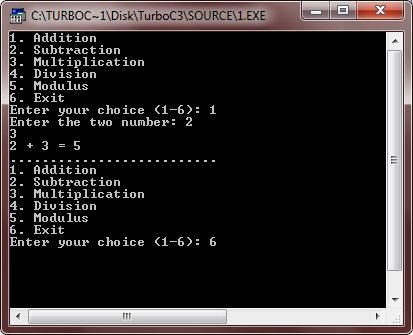# C switch Statement

The switch statement in C, is a multiple-branch selection statement which is successively tests the value of an expression against a list of character or integer constants. In this selection, when a match is found, the statements associated with this match constant are executed. Here is the syntax of switch statement :

```switch(expression)
{
case constant1 : statement sequence;
break;
case constant2 : statement sequence;
break;
case constant3 : statement sequence;
break;
.
.
.
default : statement sequence;
}```

here, if the match is found with any constant, then the statement sequence associated with that case is executed until the break statement or the end of the switch statement is reached, otherwise the statement sequence of the default will be executed. Let's take a look at the following example.

### C switch statement Example

Here is the example program that demonstrates the switch statement in C:

```#include<stdio.h>
#include<conio.h>
#include<stdlib.h>
void main()
{
int num1, num2, res;
char choice;
clrscr();

do
{
printf("2. Subtraction\n");
printf("3. Multiplication\n");
printf("4. Division\n");
printf("5. Modulus\n");
printf("6. Exit\n");

scanf("%c", &choice);

switch(choice)
{
case '1' :
printf("Enter the two number: ");
scanf("%d%d", &num1, &num2);
res = num1 + num2;
printf("%d + %d = %d",num1, num2, res);
break;

case '2' :
printf("Enter the two number: ");
scanf("%d%d", &num1, &num2);
res = num1 - num2;
printf("%d - %d = %d",num1, num2, res);
break;

case '3' :
printf("Enter the two number: ");
scanf("%d%d", &num1, &num2);
res = num1 * num2;
printf("%d * %d = %d",num1, num2, res);
break;

case '4' :
printf("Enter the two number: ");
scanf("%d%d", &num1, &num2);
res = num1/num2;
printf("%d / %d = %d",num1, num2, res);
break;

case '5' :
printf("Enter the two number: ");
scanf("%d%d", &num1, &num2);
res = num1%num2;
printf("%d %% %d = %d",num1, num2, res);
break;

case '6' : exit(0);
break;

default : printf("Wrong choice..!!\n");
}
printf("\n..........................\n");

}while(choice!=6 && choice!=getchar());

getch();
}```

Here is the sample run of the above C program:## C Nested switch statements

You can also have a switch as a part of the statement sequence of an outer switch. If the case constants of the inner and the outer switch contain the common values, no conflicts arise. Here is an example of nested switch statement:

```switch(x)
{
case 1 : switch(y)
{
case 0 : printf("Divide by zero error..!!\n");
break;

case 1 : process(x, y);
break;
}
break;

case 2 :
.
.
.
}```

### More Examples

Here are the list of some more examples that you can go for:

Tools
Calculator